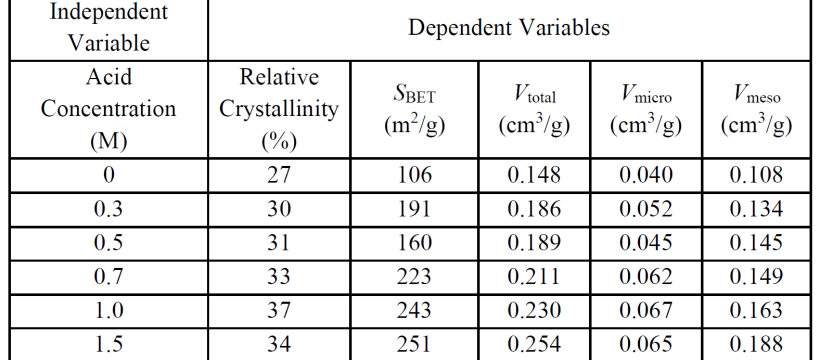# Assignment of Computer Application and Environmental Modelling (30 November 2022)1. Each student must complete the following three problems assignment on using the ORIGIN application for graphing and curve fitting as well as testing its fitness, and the STATISTICA / DESIGN EXPERT application for design of experiments, modeling and testing its fitness, optimization, and graphical presentation.
2. [PROBLEM #1] Make/Prepare a multi Y curve (YY-Y-YY) for the data in the following table:
•3. [PROBLEM #2] Make/do a Curve Fitting model that is suitable for the experimental data in the table above between: V total = f (Acid Concentration) ; please select the model that best fits the experimental data; also present the fitness test (using the Coefficient of Determination (R2) and ANOVA); provide an explanation of the interpretation of the fitness test (R2 and ANOVA).
4. [Problem #3] Using the STATISTICA / DESIGN EXPERT software, make (reproduce) a Design of Experiment for the following experimental data, starting from designing the experiment (DOE), conducting the modeling, including the fitness test (using the Coefficient of Determination (R2) and ANOVA) ; provide an explanation of the interpretation of the fitness test; as well as optimization. Provide graphs of the results in the form of surface curves, contour curves, experimental vs calculated, the regression model equations, ANOVA, and carry out the process of ignoring (ignoring) the term model that is not significant.
•5. This assignments should be collected by uploading a document file in PDF format, where the file name contains information on the Student’s Full Name and NIM). Note: You only have one chance to upload the assignment file, so make sure the assignment file is correct. URL for assignment submission here: https://bit.ly/aplikomint30nov2022
6. Late collection of assignments : Wednesday, 21 December 2022 time 04.00 pm (GMT+7).